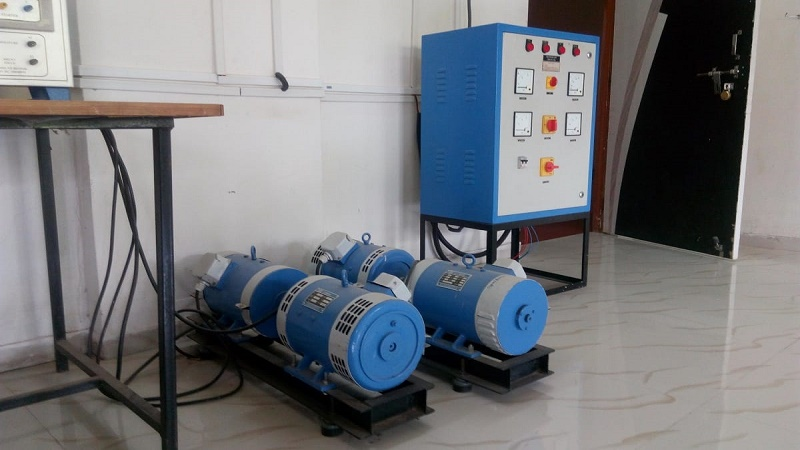Electrical Machines II - EE8401, EE6504

Online Study Material, Lecturing Notes, Assignment, Reference, Wiki and important questions and answersSYNCHRONOUS GENERATOR

=> Fundamental Principles of A.C. Machines
=> E.M.F. equation of an elementary alternator single phase
=> E.M.F. equation of an elementary alternator three phase
=> Relation between speed and frequency
=> Factors affecting the induced emf (Coil Pitch and Distributed Windings) - Synchronous Generator
=> Armature Reaction - Synchronous Generator
=> Synchronous Generators
=> Open circuit characteristic (OCC) and Short Circuit Characteristics (SCC) of a Synchronous generator
=> Armature Reaction Reactance - Synchronous Generators
=> Synchronous reactance
=> Short Circuit Ratio (SCR) - Synchronous Generator
=> Load Characteristics - Synchronous Generator
=> Potier Reactance - Synchronous Generator
=> Voltage Regulation - Synchronous Generator
=> Methods of finding Voltage Regulation in Synchronous Generator
=> Power angle characteristics
=> Salient pole alternators and Blondel's Two Reaction Theory
=> Direct-axis and Quadrature-axis Synchronous Reactances
=> Power Angle Characteristic of Salient Pole Machine
=> Slip Test
=> Parallel Operation of Alternators
=> Synchronizing Current
=> Effect of Change of Excitation
=> Effect of Change of Input Torque
=> Sharing of load when two alternators are in parallel
=> Prime-mover Governor Characteristic
=> Important Short Questions and Answers: Synchronous Generator

SYNCHRONOUS MOTOR

=> Synchronous Motors: Construction, Operating Principle, Equivalent Circuit
=> Phasor Diagram - Synchronous Motors
=> Torque and Power Relations
=> V-Curves and Inverted V-Curves
=> Effect of Changing Field Excitation at Constant Load
=> Methods of starting synchronous motor
=> Performance Characteristic of Synchronous Motors
=> Hunting and Damper Winding
=> Synchronous Induction Motor
=> Important Short Questions and Answers: Synchronous Motors

THREE PHASE INDUCTION MOTOR

=> Three Phase Induction Motor
=> Types and Construction of Three Phase Induction Motor
=> Squirrel cage Induction Motors
=> Slip ring Induction Motors
=> Principle of Operation - Three Phase Induction Motor
=> Rotating Magnetic Field and Induced Voltages
=> Alternate Analysis for Rotating Magnetic Field
=> Phasor Diagram of Three Phase Induction Motor
=> Equivalent Circuit of Three Phase Induction Motor
=> Power and Torque Relations of Three Phase Induction Motor
=> Induction Motor Torque
=> Rotor Output
=> Circle Diagram - analyse the three phase induction motor performance
=> Tests to Determine the Equivalent Circuit Parameters - Induction Motor
=> Construction of Circle Diagram - Induction Motor
=> Performance Characteristics of Three phase Induction Motor
=> Operation with Unbalanced Supply Voltage on Polyphase Induction Motors
=> Important Short Questions and Answers: Three Phase Induction Motor

STARTING AND SPEED CONTROL OF THREE PHASE INDUCTION MOTOR

=> Methods of Starting Three Phase Induction Motors
=> Starting of Slip-Ring Induction Motors
=> Speed control of Three Phase Induction Motors
=> Changing applied voltage - Speed control of Three Phase Induction Motors
=> Rotor resistance control - Speed control of Three Phase Induction Motors
=> Cascade control - Speed control of Three Phase Induction Motors
=> Pole changing method - Speed control of Three Phase Induction Motors
=> Stator frequency control - Speed control of Three Phase Induction Motors
=> Power Stages in an Induction Motor
=> Double Cage Induction Motor: Construction, Working
=> Cogging and Crawling of Induction Motor
=> Induction Generator
=> Induction Motor Braking Regenerative Plugging Dynamic
=> Important Short Questions and Answers: Starting and Speed Control of Three Phase Induction Motor

SINGLE PHASE INDUCTION MOTORS AND SPECIAL MACHINES

=> Single Phase Induction Motors
=> Double Revolving Field Theory of Single Phase Induction Motor
=> Equvalent Circuit of Single Phase Induction Motor
=> Methods of Starting Single Phase Induction Motor
=> Principle and Working of Split Phase Induction Motor
=> Types of Split-Phase Induction Motors
=> Repulsion starting - Single Phase Induction Motor: Construction, Working and Characteristics
=> Shaped Pole Starting - Single Phase Induction Motor
=> Single Phase Series Motor: Operation, Applications, Phasor Diagram
=> Schrage Motor
=> Universal Motors: Operation, Characteristic, Applications
=> Magnetic Levitation
=> Important Short Questions and Answers: Single Phase Induction Motor and Special Machines

EE6504 Electrical Machines II - Anna University 2013 Regulation Syllabus - Download Pdf
EE8401 Electrical Machines II - Anna University 2017 Regulation Syllabus - Download Pdf